# Truncated icosidodecahedron

﻿
Truncated icosidodecahedron
Truncated icosidodecahedron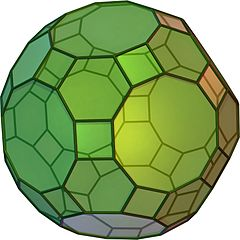Type Archimedean solid
Uniform polyhedron
Elements F = 62, E = 180, V = 120 (χ = 2)
Faces by sides 30{4}+20{6}+12{10}
Schläfli symbol t0,1,2{5,3}
Wythoff symbol 2 3 5 |
Coxeter-DynkinSymmetry Ih, [5,3], (*532)
Dihedral Angle hexagon-decagon: 142.62°
square-decagon: 148.28°
hexagon-square: 159.095°
References U28, C31, W16
Properties Semiregular convex zonohedron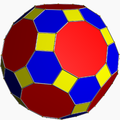Colored faces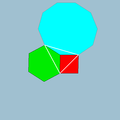4.6.10
(Vertex figure)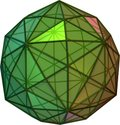Disdyakis triacontahedron
(dual polyhedron)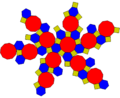Net

In geometry, the truncated icosidodecahedron is an Archimedean solid, one of thirteen convex isogonal nonprismatic solids constructed by two or more types of regular polygon faces.

It has 30 square faces, 20 regular hexagonal faces, 12 regular decagonal faces, 120 vertices and 180 edges – more than any other nonprismatic uniform polyhedron. Since each of its faces has point symmetry (equivalently, 180° rotational symmetry), the truncated icosidodecahedron is a zonohedron.

## Other names

Alternate interchangeable names include:

The name truncated icosidodecahedron, originally given by Johannes Kepler, is somewhat misleading. If one truncates an icosidodecahedron by cutting the corners off, one does not get this uniform figure: instead of squares the truncation has golden rectangles. However, the resulting figure is topologically equivalent to this and can always be deformed until the faces are regular.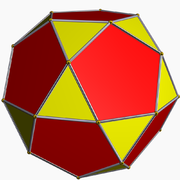Icosidodecahedron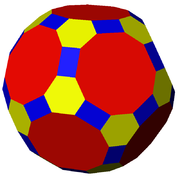A literal geometric truncation of the icosidodecahedron produces rectangular faces rather than squares.

The alternative name great rhombicosidodecahedron (as well as rhombitruncated icosidodecahedron) refers to the fact that the 30 square faces lie in the same planes as the 30 faces of the rhombic triacontahedron which is dual to the icosidodecahedron. Compare to small rhombicosidodecahedron.

One unfortunate point of confusion is that there is a nonconvex uniform polyhedron of the same name. See nonconvex great rhombicosidodecahedron.

## Area and volume

The surface area A and the volume V of the truncated icosidodecahedron of edge length a are:\begin{align} A & = 30 \left [ 1 + \sqrt{ 2 \left ( 4 + \sqrt{5} + \sqrt{15+6\sqrt{6}} \right ) } \right ] a^2 \\ & \approx 175.031045a^2 \\ V & = ( 95 + 50\sqrt{5} ) a^3 \approx 206.803399a^3. \\ \end{align}

Truncated icosidodecahedron is the largest solid among all 13 Archimedean solids with the same side lengths.

## Cartesian coordinates

Cartesian coordinates for the vertices of a truncated icosidodecahedron with edge length 2τ − 2, centered at the origin, are all the even permutations of:

(±1/τ, ±1/τ, ±(3+τ)),
(±2/τ, ±τ, ±(1+2τ)),
(±1/τ, ±τ2, ±(−1+3τ)),
(±(-1+2τ), ±2, ±(2+τ)) and
(±τ, ±3, ±2τ),

where τ = (1 + √5)/2 is the golden ratio.

## Orthogonal projections

The truncated icosidodecahedron has seven special orthogonal projections, centered on a vertex, on three types of edges, and three types of faces: square, hexagonal and decagonal. The last two correspond to the A2 and H2 Coxeter planes.

Orthogonal projections
Centered by Vertex Edge
4-6
Edge
4-10
Edge
6-10
Face
square
Face
hexagon
Face
decagon
Image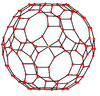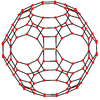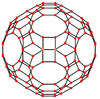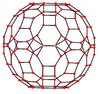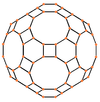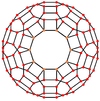Projective
symmetry
+      

## Spherical tiling

The truncated icosidodecahedron can also be represented as a spherical tiling, and projected onto the plane via a stereographic projection. This projection is conformal, preserving angles but not areas or lengths. Straight lines on the sphere are projected as circular arcs on the plane.

Wikimedia Foundation. 2010.

### Look at other dictionaries:

• Great truncated icosidodecahedron — In geometry, the great truncated icosidodecahedron is a nonconvex uniform polyhedron, indexed as U68.Cartesian coordinates Cartesian coordinates for the vertices of a great truncated icosidodecahedron centered at the origin are all the even… …   Wikipedia

• Truncated cuboctahedron — (Click here for rotating model) Type Archimedean solid Uniform polyhedron Elements F = 26, E = 72, V = 48 (χ = 2) Faces by sides …   Wikipedia

• Truncated octahedron — (Click here for rotating model) Type Archimedean solid Uniform polyhedron Elements F = 14, E = 36, V = 24 (χ = 2) Faces by sides 6 …   Wikipedia

• Truncated great icosahedron — Type Uniform star polyhedron Elements F = 32, E = 90 V = 60 (χ = 2) Faces by sides 12{5/2}+20{6} Wythoff symb …   Wikipedia

• Truncated great dodecahedron — Type Uniform star polyhedron Elements F = 24, E = 90 V = 60 (χ = −6) Faces by sides 12{5/2}+12{10} Wythof …   Wikipedia

• Icosidodecahedron — An icosidodecahedron is a polyhedron with twenty triangular faces and twelve pentagonal faces. An icosidodecahedron has 30 identical vertices, with two triangles and two pentagons meeting at each, and 60 identical edges, each separating a… …   Wikipedia

• Truncated icosahedron — The truncated icosahedron is an Archimedean solid. It comprises 12 regular pentagonal faces, 20 regular hexagonal faces, 60 vertices and 90 edges. Construction This polyhedron can be constructed from an icosahedron with the 12 vertices truncated… …   Wikipedia

• Truncated dodecahedron — In geometry, the truncated dodecahedron is an Archimedean solid. It has 12 regular decagonal faces, 20 regular triangular faces, 60 vertices and 90 edges. TOC Geometric relations This polyhedron can be formed from a dodecahedron by truncating… …   Wikipedia

• Liste Des Polyèdres Uniformes — Les polyèdres uniformes et les pavages forment un groupe bien étudié. Ils sont listés ici pour une comparaison rapide de leurs propriétés et de leurs noms de schéma variés ainsi que de leurs symboles. Cette liste inclut : tous les 75… …   Wikipédia en Français

• Liste des polyedres uniformes — Liste des polyèdres uniformes Les polyèdres uniformes et les pavages forment un groupe bien étudié. Ils sont listés ici pour une comparaison rapide de leurs propriétés et de leurs noms de schéma variés ainsi que de leurs symboles. Cette liste… …   Wikipédia en Français